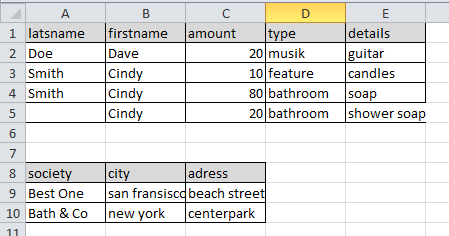# QlikView App Dev

Discussion Board for collaboration related to QlikView App Development.

Announcements
Skip the ticket, Chat with Qlik Support instead for instant assistance.
cancel
Showing results for
Did you mean:Anonymous
Not applicable

## Multiple distinct arrays on the same excel sheet

Hi everybody.

I've got an exel file to load with multiple arrays on a single sheet.

So, i would like to know if there is a solution to load each array distincly.

For exemple, Ive got this ones:I'd like to get:

tab1.firstRow = 1, tab1.lastRow = 5

tab2.firstRow = 8, tab2.lastRow = 10

(Or maybe tab2.firstRow = 6, tab2.lastRow = 8 because LOAD statement don't take the blank row)

So, I'll be able to do :

PERSON:

LET tab1.nRecords = \$(tab1.lastRow) - \$(tab1.firstRow);

LET tab1.startRow = \$(tab1.firstRow) - 1

FIRST tab1.nRecords

FROM [excel_file.xlsx]

(ooxml, embedded labels, header is tab1.startRow lines, table is sheet1);

SOCIETY:

LET tab2.nRecords = \$(tab2.lastRow) - \$(tab2.firstRow);

LET tab2.startRow = \$(tab2.firstRow) - 1

FIRST tab2.nRecords

FROM [excel_file.xlsx]

(ooxml, embedded labels, header is tab1.startRow lines, table is sheet1);

Or maybye another tip exists?

Thanks for the help !

1 Solution

Accepted SolutionsAnonymous
Not applicable
Author

Hi.

Since I've posted my question, I've worked on.

So, you can find a proposition:

// 1 - Get the start position of each array

ARRAY_STRUCTURE:

LOAD RecNo() as position, RowNo() as id, A as arrayName

FROM [excel_file.xlsx]

(ooxml, table is sheet1)

WHERE A = 'lastname' OR A = 'society';

// 2 - Create some empty tables in order to receive the data

For Each vArrayName in FieldValueList('ARRAY_STRUCTURE.arrayName')

DATA_\$(vArrayName):

AutoGenerate(0);

Next vArrayName ;

// 3 - Get data from each array (concatenate with each empty table)

FOR Each nId in FieldValueList('ARRAY_STRUCTURE.id')

LET vArrayPosition = FieldValue('ARRAY_STRUCTURE.position', nId);

LET vArrayName = FieldValue('ARRAY_STRUCTURE.position', arrayName);

LET vEndLine = FieldValue('\$(pArrayStructure).position', nId + 1) - vArrayPosition - 1;

If(Not IsNull(vEndLine)) Then

// Get data, til the next array

Concatenate(DATA_\$(vArrayName))

FIRST \$(vEndLine)

LOAD \$(nId) as tabIndex, \$(vArrayName) as arrayName, *

FROM  [excel_file.xlsx]

Else

// Last array: get all the remaining data

Concatenate(DATA_\$(vArrayName))

LOAD \$(nId) as tabIndex, \$(vArrayName) as arrayName, *

FROM [excel_file.xlsx]

EndIf;

NEXT nId ;

In order to use this method in the future, I've put each of this step in a procedure, with flexable parameters.Anonymous
Not applicable
Author

Hi.

Since I've posted my question, I've worked on.

So, you can find a proposition:

// 1 - Get the start position of each array

ARRAY_STRUCTURE:

LOAD RecNo() as position, RowNo() as id, A as arrayName

FROM [excel_file.xlsx]

(ooxml, table is sheet1)

WHERE A = 'lastname' OR A = 'society';

// 2 - Create some empty tables in order to receive the data

For Each vArrayName in FieldValueList('ARRAY_STRUCTURE.arrayName')

DATA_\$(vArrayName):

AutoGenerate(0);

Next vArrayName ;

// 3 - Get data from each array (concatenate with each empty table)

FOR Each nId in FieldValueList('ARRAY_STRUCTURE.id')

LET vArrayPosition = FieldValue('ARRAY_STRUCTURE.position', nId);

LET vArrayName = FieldValue('ARRAY_STRUCTURE.position', arrayName);

LET vEndLine = FieldValue('\$(pArrayStructure).position', nId + 1) - vArrayPosition - 1;

If(Not IsNull(vEndLine)) Then

// Get data, til the next array

Concatenate(DATA_\$(vArrayName))

FIRST \$(vEndLine)

LOAD \$(nId) as tabIndex, \$(vArrayName) as arrayName, *

FROM  [excel_file.xlsx]

Else

// Last array: get all the remaining data

Concatenate(DATA_\$(vArrayName))

LOAD \$(nId) as tabIndex, \$(vArrayName) as arrayName, *

FROM [excel_file.xlsx]